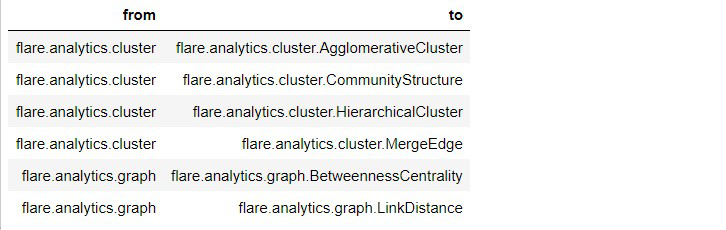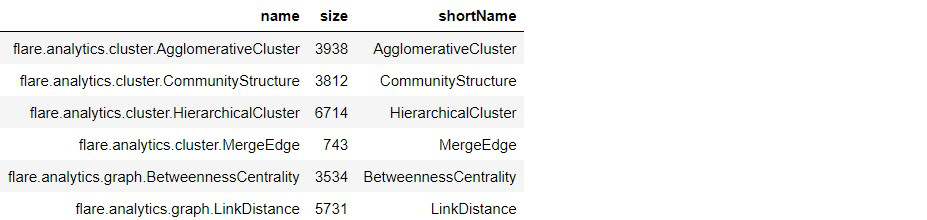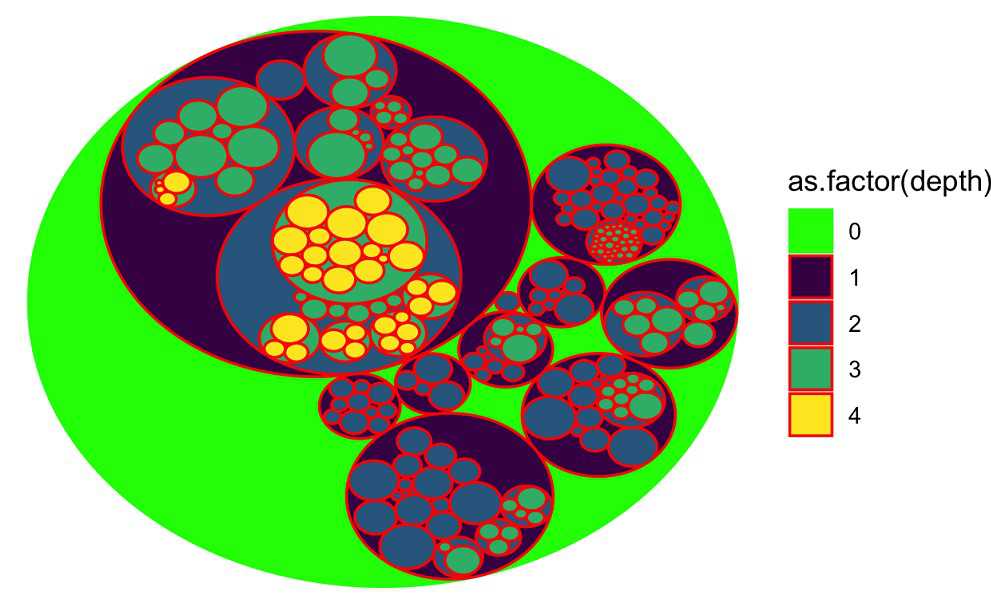# Circular Packing to visualise hierarchy data in R

• Last Updated : 16 Feb, 2022

In this article, we are talking about handling hierarchical data using circular packing visualizations. To prepare circular packing with R Programming Language, we will use ggraph package and prepare a bubble to show the hierarchies.

## Circular Packing to visualize hierarchy data in R

### Preparing the Hierarchical Data

Here we are going to prepare hierarchical data for demonstration. For this, we will use flare datasets.

## R

 `# Libraries` `library``(ggraph) ``# to prepare visualisation` `library``(igraph) ``# for network analysis` `library``(tidyverse) ``# for data handling` `library``(viridis) ``# for generating the color map`   `# data for hierarchical structure` `edges = flare\$edges` `head``(edges)`

Output:Creating another dataframe for hierarchical structure

## R

 `vertices = flare\$vertices` `head``(vertices)`

Output:Preparing the graph with dataframe:

## R

 `# preparing the graph` `mygraph <- ``graph_from_data_frame``( edges, ` `                                  ``vertices = vertices )` `mygraph`

Output:

```IGRAPH 6e05b59 DN-- 252 251 --
+ attr: name (v/c), size (v/n), shortName (v/c)
+ edges from 6e05b59 (vertex names):
 flare.analytics.cluster->flare.analytics.cluster.AgglomerativeCluster
 flare.analytics.cluster->flare.analytics.cluster.CommunityStructure
 flare.analytics.cluster->flare.analytics.cluster.HierarchicalCluster
 flare.analytics.cluster->flare.analytics.cluster.MergeEdge
 flare.analytics.graph  ->flare.analytics.graph.BetweennessCentrality
 flare.analytics.graph  ->flare.analytics.graph.MaxFlowMinCut
 flare.analytics.graph  ->flare.analytics.graph.ShortestPaths
+ ... omitted several edges```

### Visualize Circular Hierarchy

Here we will visualize the dataframe with a hierarchical structure.

## R

 `# plot the graph using ggraph` `ggraph``(mygraph, ``# graph data` `       ``layout = ``'circlepack'``,` `       `  `       ``# size of bubbles based on` `       ``# the size parameter in vertices data` `       ``weight = size) + ` `  ``geom_node_circle``(``aes``(fill = ``as.factor``(depth), ` `                       ``color = ``as.factor``(depth) )) +` `  ``# define the color of each different labels` `  ``scale_color_manual``( values=``c``(``"0"` `= ``"green"``, ``"1"` `= ``"red"``,` `                               ``"2"` `= ``"red"``, ` `                               ``"3"` `= ``"red"``, ``"4"``=``"red"``) ) +` `  ``scale_fill_manual``(values = ``c``(``"0"` `= ``"green"``, ``"1"` `= ``viridis``(4), ` `                               ``"2"` `= ``viridis``(4), ``"3"` `= ``viridis``(4), ` `                               ``"4"` `= ``viridis``(4))) + ` `  ``theme_void``()`

Output:My Personal Notes arrow_drop_up
Recommended Articles
Page :